Motion of Spinning Eggs

Ko Munakata October 31, 2007

(Submitted as coursework for Physics 210, Stanford University, Autumn 2007)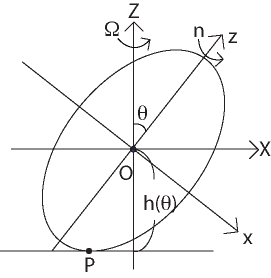Fig. 1: The definition of coodinates and notation used in this calculation.

Introduction

If you rapidly spin a hard boiled egg which was initially lying on a table, you will see that the center of mass of the egg rises, letting its high symmetry axis stand in the direction perpendicular to the plane of the table. This phenomenon seems to contradict the law of gravity at first glance.

In this report, we describe how it can be unambiguously explained by the laws of classical mechanics . Our strategy is as follows: first we construct the Euler's angular momentum equation of the egg; next, we use several approximations in order to simplify the Euler's equation, which allows us to analytically obtain an approximate constant of motion of the system; utilizing this constant of motion then yields a simple differential equation for the angle which represents the orientation of the egg; and finally we demonstrate that the egg really rises by analytically solving the differential equation, assuming for simplicity that the egg is a uniform spheroid.

Through this procedure, we will see that the very mechanism of the rising motion of the egg is the friction between the egg and the table.

Derivation of the Euler's Equation for the Rotating Egg

We use the same geometry and notation as in , which is summerized in Fig. 1. The rotating frame OXYZ shown below is adopted to construct Euler's equation of motion .

First we need the form of angular momentum to construct the Euler's equation. In the frame Oxyz (which is rotated by the angle θ with respect to the OXYZ frame), the moment of inertia I' is diagonal with I'xx = I'yy = A and I'zz = C. The angular velocity also assumes a simple form in this frame;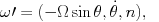(1)

where n(t) represents the angular velocity about the Oz axis and Ω represents the angular velocity for the precession of the body along OZ. Thus, in this frame the angular momentum H' is calculated to be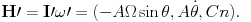(2)

Consequently, the angular momentum in the frame OXYZ can be derived by using a rotation matrix, resulting in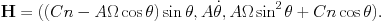(3)

Next, we consider the torque exerted to the egg because it is also needed to write down the Euler's equation. The torque exerted on the egg originates from two forces: a normal reaction force from the table R = (0, 0, R) and a frictional force F = (FX, FY, 0), both at the point P at which the egg touches the table. Note that R is of the same order of gravitational force Mg.

On the other hand, we also need to calculate the vector from O to P in order to obtain the torque. This vector is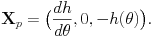(4)

Although here we do not show the reason why the X- and Z-component of XP are related with each other in this way, it can be proved by the methods of ordinary calculus.

We are now equipped with the sufficient information to construct Euler's equation of motion, which is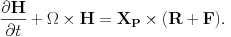(5)

Each component of the equation is explicitly shown below: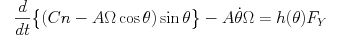(6)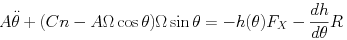(7)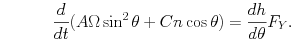(8)

Approximations and Integration of Euler's equation

The Euler's equation obtained above is completely general and hence quite complex; we therefore have to rely on several approximations. The approximations we hereafter adopt are very strong, making it possible to explicitly integrate the equation and give a simple constant of motion. These approximations are also very convenient, or almost necessary, to highlight the particular physical situation of our interest.

First, we observe in the Euler's equation above that in a general situation the time derivative of θ must have both slow and fast components. The slow component represents long time-scale rising motion of the egg, while the fast component corresponds to the rotation motion of the egg with the time-scale comparable to Ω and n. However, what we experimentally do in order to observe the rising motion of a boiled egg is to rotate the egg such that the long time-scale rising motion is readily observable; this condition allows us to argue that the time derivative of θ does not have the fast component in the situation of our interest. We therefore assume that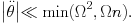(9)

This approximation is used to ignore the second time derivative of θ in the Y-component of the Euler's equation (7).

The second approximation is to further simplify (7). Here we assume that the terms which include Ω are much larger than the right-hand side of (7), which, as we see, consist of terms about a friction and a normal reaction. The physical meaning of this approximation is obvious: we rotate the egg so quickly that it can overcome any frictional or gravitational force. This approximation also reflects an actual experimental requirement because the egg in fact does not rise unless the initial velocity is fast enough.

These approximations greatly simplify eq. (7) and we immediately see that whenever sin(θ) ≠ 0,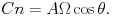(10)

Next we substitute this simple relation into the X- and Z-component of the Euler's eqs. (6) and (8), yielding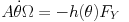(11)

and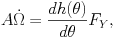(12)

respectively. Eliminating FY from these equations gives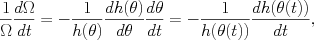(13)

which we can easily integrate to obtain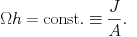(14)

J is obviously a constant of motion in this problem and is therefore determined only by initial conditions.

Note that this last relation (14) already suggests a qualitative answer to our original question "why rotating eggs can rise?". The reason is as follows. The initial rapid angular velocity Ω must gradually decrease due to a frictional force. This in turn means that h(θ) increases due to the existence of the constant J, hence the egg rises! The crucial observation here is that we need a mechanism to make Ω slower and slower as time goes by, which is in our case friction.

Derivation of the Differential Equation for θ

Now that we have already obtained a constant of motion, the rest of the problem is very simple. We just substitute (14) into (11) and get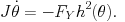(15)

If the functional forms of h(θ) and FY are determined, θ is thus to be calculated.

This differential equation explicitly demonstrates that the qualitative consideration above, which predicts that friction is the very source of the rising motion, is correct. If the table had no friction, the equation would force θ to be constant and no rise would occur.

Example of Analytic Solutions for Simple Cases

In this section, let us explicitly confirm the "rise" motion by analytically solving the above equation for simple cases. To do so, we first assume simple shape of the egg, that is, a uniform spheroid defined as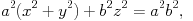(16)

where a > b (as in Fig. 1). In this case, we can easily calculate A, C and h(θ) as follows: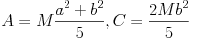(17)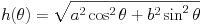(18)

or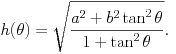(19)

In addition, we use two different forms for the friction FY.

FY Constant (Coulomb's Law of Friction)

In this case, all we need to do is to substitute (18) into (15) to obtain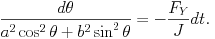(20)

Both sides of this equation can be explicitly integrated and we have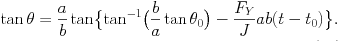(21)

Note that since friction should act in the opposite direction from the velocity of the point P, FY should have the same sign as Ω when θ is positive, as is easily verified. According to (14), this means that FY /J is positive and hence (21) suggests decrease of θ as t increases.

Substitution of (21) into (19) yields the explicit functional form of h(θ(t)), which is plotted in Fig. 2.

FY Proportional to Velocity (Viscous Law of Friction)

In this case, we first have to derive the velocity of the point P. Since the angular velocity of the body is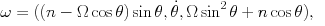(22)

which can be derived by rotating ω' by the angle θ (see (1)), the velocity of the point P is written as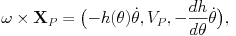(23)

where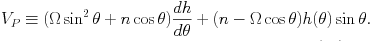(24)

By eliminating Ω and n using (10) and (14), VP is rewritten as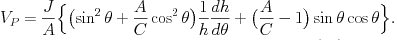(25)

Furthermore, the simple geometry specified by (16)-(19) greatly simplifies (25), giving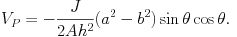(26)

Assuming that FY is proportional to VP, we have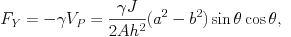(27)

and eq. (15) now becomes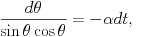(28)

where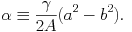(29)

This equation is again analytically integrable, resulting in the following final expression: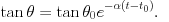(30)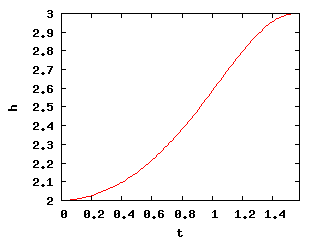Fig. 2: The height of the center of mass h as a function of time (derived from (19) and (21)) when the Coulomb law of friction is adopted. We used a = 3.0, b = 2.0, FYab/J = 1.0, t0 = 0.0 and θ0 = π/2 - 0.01.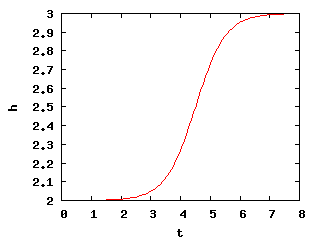Fig. 3: The height of the center of mass h as a function of time (derived from (19) and (30)) when the viscous law of friction is adopted. We used a = 3.0, b = 2.0, α = 1.0, t0 = 0.0 and θ0 = π/2 - 0.01.

By substituting (30) into (19), we obtain h(θ(t)), which is plotted in Fig. 3. Comparing Fig. 2 and Fig. 3 suggests that the two forms of frictional force do not have qualitatively big difference. One small difference is that in the case of Coulomb's law θ actually becomes 0 within finite time interval, at which we cannot use the relation (10) any more. Physical intuition suggests that in this case θ precesses around 0. On the other hand, with the viscous law of friction θ keeps on approaching to 0 asymptotically, but never reaches there. I speculate that since we already oversimplified the problem by the approximation (9), it may not be a good idea to determine which frictional force is more physical from the argument above. Numerical simulation seems to be necessary to examine the problem in that detail.

© 2007 Ko Munakata. The author grants permission to copy, distribute and display this work in unaltered form, with attributation to the author, for noncommercial purposes only. All other rights, including commercial rights, are reserved to the author.

References

 H. K. Moffatt and Y. Shimomura, Nature 416, 385 (2002).

 H. Goldstein, C. Poole and J. Safko, Classical Mechanics (Addison-Wesley, 2002).